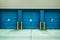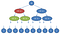# Merge Sort Algorithm

## Divide and Conquer RecursionPhoto by Steve Johnson on Unsplash

## PsuedoCode

`PROCEDURE MERGESORT(ARRAY)  IF(ARRAY.LENGTH == 1) THEN    RETURN ARRAY  ELSE IF(ARRAY.LENGTH ==2) THEN    RETURN [MIN(ARRAY),MAX(ARRAY)]  ELSE    RETURN COMBINE(       MERGESORT([ARRAY...ARRAY[length/2]]),        MERGESORT([...ARRAY[length/2 + 1]])) END IFPROCEDURE COMBINE(ARRAYA,ARRAYB)  LOOP THROUGH LARGERARRAY    IF ARRAYA[i] < ARRAYB[i] THEN      PUSH ARRAYA[i] > EMPTYARRAY    ELSE      PUSH ARRAYB[i] > EMPTYARRAY    ENDIF  RETURN COMBINEARRAYEND PROCEDUREEND PROCEDURE`

Looking at the pseudocode above, we can see that the algorithm consists of two important sections. First, an ‘if-else’ conditional branch is combined with a recursive call. Second, this recursive call is helped with a ‘helper’ procedure that combines two previous sorted arrays.

The basic logic flows as follows:

1. If the array length is one, return the array.
2. If the array length is two, return the sorted array by simply swapping the min value and maximum value.
3. If the array length is larger than two, split the array in half.
4. Then recursively call merge sort on each of those halves.
5. On the return of these recursive calls, combine the two already sorted half arrays.
6. As the recursive calls return from the stack, the eventual product is a fully sorted array.

## Implementation

`//main mergeSort function-implements top down recursive approachfunction mergeSort (unsortedArray) {  //return array back if length is 1    if (unsortedArray.length <= 1) {      return unsortedArray;  //return [min,max] if array has only two elements    } else if(unsortedArray.length <= 2) {     return [Math.min.apply(null,unsortedArray),           Math.max.apply(null,unsortedArray)]   }  //if array has more than two elements it must be split  //first find middle point  const middle = Math.floor(unsortedArray.length / 2);  const left = unsortedArray.slice(0, middle);  const right = unsortedArray.slice(middle);// With the array now split in two recursively call merge Sort and //combine results  return merge(mergeSort(left),mergeSort(right));}//Merge combines reults of two merge sortsfunction merge (left, right) {  let resultArray = [], leftIndex = 0, rightIndex = 0;while (leftIndex < left.length && rightIndex < right.length) {    if (left[leftIndex] < right[rightIndex]) {      resultArray.push(left[leftIndex]);      leftIndex++;    } else {      resultArray.push(right[rightIndex]);   rightIndex++;    }  }return [...resultArray, ...left.slice(leftIndex),...right.slice(rightIndex)]}`

The JavaScript implementation uses a mergeSort function that handles most of the logic coordination and a “helper” function that combines already sorted arrays. The flow of the program is illustrated below with an example array: [7,3,5,8,13,1,2,99,9,4].

## Time and Space ComplexityWikipedia Commons

Merge Sort’s time complexity is determined by the number of comparisons that occur through the algorithm. Like most “divide and conquer” algorithms, one of its terms is logarithmic. Looking at the tree diagram above, we can see that the worst-case n comparisons are made at every tree level. Each level represents a finer division of the number list. Therefore the time complexity will be n times the height of the binary tree above. Consequently, the final time complexity is:

`f(n)=O(n(ln(n))), where n is the size of input array`

The algorithm does require the creation of two sub-arrays in addition to the original array. This is necessary for the recursive calls to work properly. Consequently, the algorithm must create n elements in memory. Thus the space complexity is O(n).

## Key TakeAways

1. It uses a divide and conquer method. This method can be implemented bottom-to-up recursively or top-to-bottom with a loop.
2. The Merge Sort algorithm has a space and time complexity of O(n) and O(n ln n).

## CodeX

Everything connected with Tech & Code

Written by

## Adam Shaffer

A full-stack software developer who likes writing about tech.

Everything connected with Tech & Code

Written by

## Adam Shaffer

A full-stack software developer who likes writing about tech.

Medium is an open platform where 170 million readers come to find insightful and dynamic thinking. Here, expert and undiscovered voices alike dive into the heart of any topic and bring new ideas to the surface. Learn more

Follow the writers, publications, and topics that matter to you, and you’ll see them on your homepage and in your inbox. Explore

If you have a story to tell, knowledge to share, or a perspective to offer — welcome home. It’s easy and free to post your thinking on any topic. Write on Medium